The Art of Interface

# Fast Fourier transform — FFT

Category. Digital signal processing (DSP) software development.

Abstract. The article is a practical tutorial for fast Fourier transform — FFT — understanding and implementation. Article contains theory, C++ source code and programming instructions. Popular Cooley-Tukey technique is considered.

References. Case study: ECG processing — R-peaks detection and 4D ultrasound volume image processing.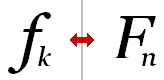## 1. Introduction to fast Fourier transform

Fast Fourier transformFFT — is speed-up technique for calculating discrete Fourier transformDFT, which in turn is discrete version of continuous Fourier transform, which indeed is origin for all its versions. So, historically continuous form of the transform was discovered, then discrete form was created for sampled signals and then algorithm for fast calculation of discrete version was invented.

## 2. Understanding FFT

First of all let us have a look at what Fourier transform is. Fourier transform is an integral of the form: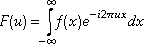(1)

The transform operates in complex domain. Recall, that imaginary exponent could be written as:(2)

For sampled function continuous transform (1) turns into discrete one: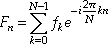(3)

Expression (3) is discrete Fourier transformDFT. Here {f0, f1, ... , fN−1} is input discrete function and {F0, F1, ... , FN−1} is result of Fourier transform.

It is easily could be seen that to program DFT it is enough to write double loop and just calculate sums of products of input samples and imaginary exponents. The number of operations required is obviously of O(N2) order. But due to transform properties it is possible to reduce the number of operations to the order of O(Nlog2N). Now, let us explore tricks we can use to speed-up calculations.

Let us put N=8 and write down our DFT: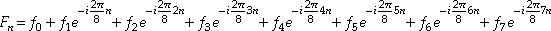(4)

Easily could be seen we can split the sum into two similar sums separating odd and even terms and factoring out the latter sum:(5)

Now we can split the sums in brackets again: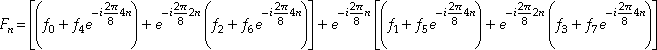(6)

Thus, we have 3 — log28 — levels of summation. The deepest one in parenthesis, the middle one in brackets and the outer one. For every level exponent multiplier for the second term is the same.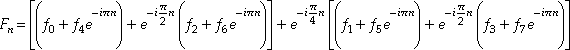(7)

And now the most important observation one can make to get speed-up: periodicity of the exponent multipliers.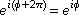(8)

For the exponent multiplier in parenthesis period is n=2, which means sums in parenthesis are exactly the same for n=0, 2, 4, 6 and for n=1, 3, 5, 7. It means on deepest level in parenthesis we need 4×2=8 — number of sums times period — sums in total. And note, since n=1, 3, 5, 7 corresponds to the half of the period π, exponent multiplier is the same as for n=0, 2, 4, 6 but with the opposite sign.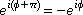(9)

Indeed they are 1 for n=0, 2, 4, 6 and −1 for n=1, 3, 5, 7.

For the exponent multiplier in brackets period is n=4, which means we have the same sums for pairs n=0, 4; n=1, 5; n=2, 6 and n=3, 7. It means on the middle level in brackets we have 2×4=8 sums and the second half of them could be received again by changing sign in first half of them — due to the fact distance between n and n+2 is π. Thus, for n=0, 4 the factor is 1 and for n=2, 6 it is −1; for n=1, 5 it equals −i and for n=3, 7 it is i.

On the outer level we have just one sum for every transform component, and the period of the exponent multiplier is 8. Which gives us 1×8=8 sums and second half of them could be received by changing sign in the first half.

So, on every calculation level we have 8 sums. In terms of N it means we have log2N levels and N sums on every level, which gives us O(Nlog2N) order of number of operations. On the other hand having the constant number of sums on every level means we can process data in-place.

In summary, we have got fast Fourier transformFFT. Now it is time to develop step-by-step instruction list to be carved in code.

## 3. FFT algorithm

Having understanding of what features of DFT we are going to exploit to speed-up calculation we can write down the following algorithm:

1. Prepare input data for summation — put them into convenient order;
2. For every summation level:
1. For every exponent factor of the half-period:
1. Calculate factor;
2. For every sum of this factor:
1. Calculate product of the factor and the second term of the sum;
2. Calculate sum;
3. Calculate difference.

First step of reordering is putting input data into their natural order in (7): {f0, f1, f2, f3, f4, f5, f6, f7}→{f0, f4, f2, f6, f1, f5, f3, f7}. Since this new order was received as result of successive splitting terms into even and odd ones, in binary domain it looks like ordering based on bit greatness starting from the least significant bit — see table 1.

 0 000 000 000 0 1 001 010 100 4 2 010 100 010 2 3 011 110 110 6 4 100 001 001 1 5 101 011 101 5 6 110 101 011 3 7 111 111 111 7
Table. 1. Reordering in binary domain.

This leads to “mirrored arithmetics” — result binary column in the mirror looks like {0, 1, 2, 3, 4, 5, 6, 7} — our initial order indeed. Thus, to reorder input elements it is enough just to count in mirrored manner. Since double mirroring gives again the same number, reordering reduces to swaps.

Summation levels include our parenthesis, brackets and outer level. In general case this leads to iterations on pairs, quadruples, octads and so on.

Further, we iterate on components of half-period, second half-period we are getting as result of taking differences instead of sums for the first half. Thus, for the deepest level of parenthesis period is 2 and half-period is 1, which means this cycle will be executed only once. For the second level period is 4 and half-period is 2 and cycle will be executed 2 times. In general case we have succession 1, 2, 4, 8, ... for this cycle.

Factor calculation is calculation of our imaginary exponent. To restrict the number of trigonometric function calls (2) we use the recurrence: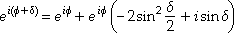(10)

Which is indeed expression(11)

written in a tricky way not to lose significance for small δ — for this case cosδ≈1 and sinδδ, which tells us that for cosδ memory will be just packed with .999999(9) but for sinδ there will be much more useful information. Thus, (10) is just the way to eliminate cosδ from calculations. If you look back at (7) you will find, that for N=8 δ=π/2, π/4 — not a small numbers. But for transforms of much bigger N δ=π/2, π/4, π/8, ... up to 2π/N, for sure, could be very small.

The innermost loop looks for sums, where calculated imaginary exponent present, calculates product and takes sum and difference, which is the sum for the second half-period at π distance, where our exponent changes its sign but not the absolute value according to (9). To perform in-place processing we utilize the following scheme: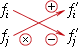Fig. 1. FFT butterfly.

This operation is elementary brick of in-place FFT calculation and usually is called butterfly. The bottom term is multiplied by imaginary exponent and then sum of the terms is stored in place of the upper term and difference is stored in place of the bottom term. General butterfly picture is depicted below — fig. 2.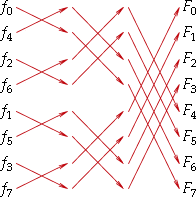Fig. 2. All FFT butterflies for N=8.

Elegant scheme. It is time to engrave this beauty in code. But before we delve into programming let us make a small digression: it is known thing that Fourier transform is a transfer to frequency domain, so, let us see how to be back from the realm of frequencies.

## 4. Inverse Fourier transform

Expression for inverse Fourier transform is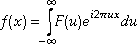(12)

and its discrete counterpart is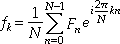(13)

Thus, the difference between forward (3) and inverse (13) transforms is just a sign and not necessary scale factor — one does not need it if interested in ratio between components but not in absolute values. It means that the routine for forward transform with slight modification can perform inverse one as well.

## 5. FFT programming

Here is our FFT class declaration.

``````class CFFT
{
public:
//   FORWARD FOURIER TRANSFORM
//     Input  - input data
//     Output - transform result
//     N      - length of both input data and result
static bool Forward(const complex *const Input, complex *const Output,
const unsigned int N);

//   FORWARD FOURIER TRANSFORM, INPLACE VERSION
//     Data - both input data and output
//     N    - length of both input data and result
static bool Forward(complex *const Data, const unsigned int N);

//   INVERSE FOURIER TRANSFORM
//     Input  - input data
//     Output - transform result
//     N      - length of both input data and result
//     Scale  - if to scale result
static bool Inverse(const complex *const Input, complex *const Output,
const unsigned int N, const bool Scale = true);

//   INVERSE FOURIER TRANSFORM, INPLACE VERSION
//     Data  - both input data and output
//     N     - length of both input data and result
//     Scale - if to scale result
static bool Inverse(complex *const Data, const unsigned int N,
const bool Scale = true);

protected:
//   Rearrange function and its inplace version
static void Rearrange(const complex *const Input, complex *const Output,
const unsigned int N);
static void Rearrange(complex *const Data, const unsigned int N);

//   FFT implementation
static void Perform(complex *const Data, const unsigned int N,
const bool Inverse = false);

//   Scaling of inverse FFT result
static void Scale(complex *const Data, const unsigned int N);
};``````

Class has four public methods for performing FFT: two functions for forward transform and two ones for inverse transform. Every couple consists of in-place version and version that preserves input data and outputs transform result into provided array.

Protected section of the class has as well four functions: two functions for data preprocessing — putting them into convenient order, core function for transform performing and auxiliary function for scaling the result of inverse transform.

Every of four public methods is very similar and is indeed wrapper that controls processing workflow. Let us see how one of them designed.

``````//   FORWARD FOURIER TRANSFORM, INPLACE VERSION
//     Data - both input data and output
//     N    - length of both input data and result
bool CFFT::Forward(complex *const Data, const unsigned int N)
{
//   Check input parameters
if (!Data || N < 1 || N & (N - 1))
return false;
//   Rearrange
Rearrange(Data, N);
//   Call FFT implementation
Perform(Data, N);
//   Succeeded
return true;
}``````

Inside wrapper you can find check of input parameters, then data preparation — rearrangement, — and transform itself. `Rearrange` function uses our “mirrored mathematics” to define new position for every element and swaps elements:

``````//   Inplace version of rearrange function
void CFFT::Rearrange(complex *const Data, const unsigned int N)
{
//   Swap position
unsigned int Target = 0;
//   Process all positions of input signal
for (unsigned int Position = 0; Position < N; ++Position)
{
//   Only for not yet swapped entries
if (Target > Position)
{
//   Swap entries
const complex Temp(Data[Target]);
Data[Target] = Data[Position];
Data[Position] = Temp;
}
//   While bit is set
while (Target & (Mask >>= 1))
//   Drop bit
//   The current bit is 0 - set it
}
}``````

While loop implements addition of 1 in mirrored manner to get target position for the element.

Now there is turn of the core method, which performs our fast Fourier transform.

``````//   FFT implementation
void CFFT::Perform(complex *const Data, const unsigned int N,
const bool Inverse /* = false */)
{
const double pi = Inverse ? 3.14159265358979323846 : -3.14159265358979323846;
for (unsigned int Step = 1; Step < N; Step <<= 1)
{
const unsigned int Jump = Step << 1;
//   Angle increment
const double delta = pi / double(Step);
//   Auxiliary sin(delta / 2)
const double Sine = sin(delta * .5);
//   Multiplier for trigonometric recurrence
const complex Multiplier(-2. * Sine * Sine, sin(delta));
//   Start value for transform factor, fi = 0
complex Factor(1.);
//   Iteration through groups of different transform factor
for (unsigned int Group = 0; Group < Step; ++Group)
{
//   Iteration within group
for (unsigned int Pair = Group; Pair < N; Pair += Jump)
{
//   Match position
const unsigned int Match = Pair + Step;
//   Second term of two-point transform
const complex Product(Factor * Data[Match]);
//   Transform for fi + pi
Data[Match] = Data[Pair] - Product;
//   Transform for fi
Data[Pair] += Product;
}
//   Successive transform factor via trigonometric recurrence
Factor = Multiplier * Factor + Factor;
}
}
}``````

The code is exact reflection of our FFT algorithm and butterfly scheme in fig. 2. Difference between forward and inverse transforms is reflected in the first line of the method where the proper sign for exponent argument is set. Initializations inside outer loop are just preparations for successive calculation of factors via trigonometric recurrence. And the job done inside inner loop, which performs butterfly operations. Trigonometric recurrence in the last line is exactly our expression (10).

Wrappers for inverse transform are designed the same way as for forward one:

``````//   INVERSE FOURIER TRANSFORM, INPLACE VERSION
//     Data  - both input data and output
//     N     - length of both input data and result
//     Scale - if to scale result
bool CFFT::Inverse(complex *const Data, const unsigned int N,
const bool Scale /* = true */)
{
//   Check input parameters
if (!Data || N < 1 || N & (N - 1))
return false;
//   Rearrange
Rearrange(Data, N);
//   Call FFT implementation
Perform(Data, N, true);
//   Scale if necessary
if (Scale)
CFFT::Scale(Data, N);
//   Succeeded
return true;
}``````

The only difference is conditional scaling of the result at postprocessing stage. By default the scaling is performed according to (13) but if one interested only in relative values she can drop the corresponding flag to skip this operation. Scaling itself is a primitive function below.

``````//   Scaling of inverse FFT result
void CFFT::Scale(complex *const Data, const unsigned int N)
{
const double Factor = 1. / double(N);
//   Scale all data entries
for (unsigned int Position = 0; Position < N; ++Position)
Data[Position] *= Factor;
}
``````

Full file listings are available online as well:

Optimized for high performance source code of complex number class you can find here:

## 6. How to use

To utilize the FFT you should include header file fft.h and place in your code lines like:

``````...Usually at the top of the file
#include "fft.h"
.
...Some code here
.
//   Allocate memory for signal data
complex *pSignal = new complex;
...Fill signal array with data
//   Apply FFT
CFFT::Forward(pSignal, 1024);
...Utilize transform result
//   Free memory
delete[] pSignal;``````

Here in-place forward Fourier transform performed for signal of 1024 samples size.

## 7. Final remarks

The FFT algorithm implemented in literature is called Cooley-Tukey. There is also Sand-Tukey algorithm that rearranges data after performing butterflies and in its case butterflies look like ours in fig. 2 but mirrored to the right so that big butterflies come first and small ones do last.

From all our considerations follows that length of input data for our algorithm should be power of 2. In the case length of the input data is not power of 2 it is good idea to extend the data size to the nearest power of 2 and pad additional space with zeroes or input signal itself in a periodic manner — just copy necessary part of the signal from its beginning. Padding with zeroes usually works well.

If you were attentive you could notice that butterflies in parenthesis and brackets in (7) do not really need multiplications but only additions and subtractions. So, optimizing two deepest levels of butterflies we can even improve FFT performance.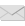Write to the author of the article — Sergey Chernenko